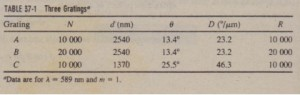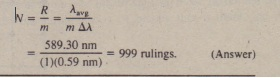# Dispersion and Resolving Power Compared

Dispersion and Resolving Power Compared

The resolving power of a grating must not be confused with its dispersion.  shows the characteristics of three gratings, all illuminated with light of wave lengthA = 589 nm, whose diffracted light is viewed in the first order  You should’ verify that the values of D and R as given in the table can be calculated with-29. respectively. (In the calculations for D. you will need to convert radians per meter to degrees per micrometer.) For the conditions noted in Table 37-1, gratings   A and B have the same dispersion and A and C have the same resolving power.   shows the intensity patterns (also called line shapes) that would be produced by these gratings for two lines of wavelengths A) and A2′ in the vicinity of A = 589 nm. Grating B, with the higher resolving power, produces narrower lines and thus is capable of distinguishing lines that are much closer together in wavelength than those in the   figure. Grating C. with the higher dispersion. produces the greater angular separation between the lines.Sample Problem

A diffraction grating has 1.26 X 104 rulings uniformly spaced over width w = 25.4 mm. It is illuminated at normal incidence by yellow  light from a sodium vapor lamp. This light contains two closely spaced emission lines (known as the sodium doublet) of wavelengths 589.00 nm and 589.59 “(a) At what angle does the first-order maximum occur (on either  side of the -nter of the diffraction pattern) for the wavelength of 589.00

SOLUTION: The Key Idea here is that the maxima produced by the  diffraction grating can be located with  (d sin () = mAl.The grating spacing d for this diffraction grating isib) Using the dispersion of the grating, calculate the angular separation between the two lines in the first order.
SOLUTION: One Key Idea here is that the angular separation /!;.() between the two lines in the first order depends on their wavelength difference A and the dispersion D of the grating, according to   (D = A). A second Key Idea is that the dispersion D  depends on the angle () at which it is to be evaluated. We can assume that, in the first  order. the two sodium lines occur close enough to each other for us to evaluate D at the angle () = 16.99° we found in part (a) for one of those lines. the dispersion as(c) What is the least number of rulings a grating can have and still be able 10 resolve the sodium doublet in the first order?

SOLUTION: One Key Idea here is that the resolving power of a grating in any order m is physically set by the number of rulings N in the  grating according to Eq. 37-29 (R = Nm). A second Key Idea is that the least wavelength difference .A that can be resolved depends on the average wavelength involved and the resolving power R of the grating. according to Eq. 37·28 (R = Aa,gI  :U). For the sodium doublet to be barely resolved, /!;.A must be their wavelength separation  of 0.59 nrn, and Aavgmust be their average wavelength of589.30 nm. Putting these ideas together, we find that the least number of rulings for a grating to resolve the sodium doublet is